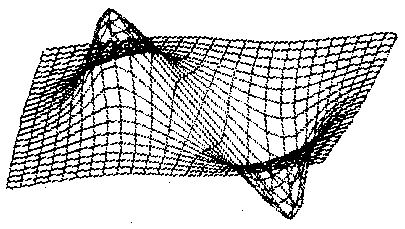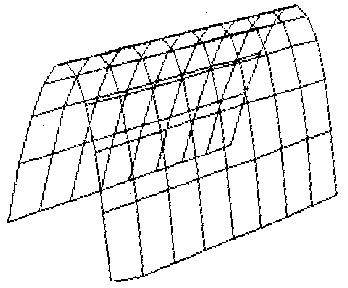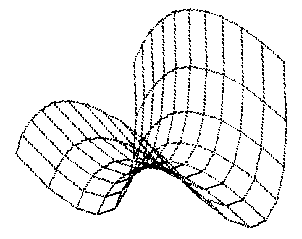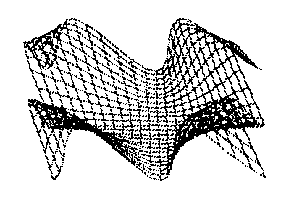`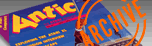ANTIC VOL. 4, NO. 6 / OCTOBER 1985`

# GRAPH 3D

## Spectacular three-dimensional visuals!

by PAUL CHABOT

Explore the world of visual calculus and create three-dimensional graphs from mathematical functions. This BASIC program will run on all Atari computers with 48K memory and a disk drive.

If you've seen the fantastic, computer-generated landscapes in movies such as "The Last Starfighter," you know what a three-dimensional graph looks like. Graph 3D creates three-dimensional objects in Graphics Mode 8, based upon functions of the form: Z = f(X,Y). Your landscapes, or objects will not be solid, but you can alter the density of the graph lines.
The second part of this article will cover technical details, but you don't need a degree in calculus to create shapes with Graph 3D. Here's how to get it up and running.

GETTING STARTED
Type in Listings 1 and 2 and check them with TYPO II. There's some machine language in these programs, so make sure that your TYPO II codes are correct and that you haven't left out any lines. SAVE Listing 1 as GR3DLOAD.BAS. Since Listing 1 automatically runs Listing 2, be sure and SAVE Listing 2 as GRAPH3D.BAS and don't try to run GRAPH3D.BAS by itself. Those using Antic's DISKIO (January, 1985) should boot these programs without it.
Run the program GR3DLOAD.BAS. First a title and then a menu will appear. After a brief pause, a black graphics screen will take its place and begin drawing a sample graph. When the graph is completed, the menu will retum. You can switch between the menu and the graphics screen by pressing [SPACE]. The commands on the menu are single letter options which can be used from either the screen or the menu. Choose a command option by pressing the letter with no [RETURN].
For example, let's SAVE the picture that's currently on the screen. Be sure there's a disk with 62 free sectors in Drive 1. Type  and, at the prompt, type in the filename and then press [RETURN] and answer [Y]. You don't need the D: device prefix and Graph 3D will automatically add an extender of .G3D. For the technically oriented, Graph 3D files are straight Graphics 8 + 16 picture files consisting of 7680 bytes of screen data followed by 13 records contaming parameter information. (See lines 910-918.)

ENTERING A FUNCTION
Let's type in a function without worrying about why it works. (We'll save that for later.) Get to the menu by pressing [SPACE], if you're not already there. Type [F] for function. You should see the current function and under it, Z =. Now type 1-2 * Y * Y and press [RETURN]. Next, type [E] for eye placement, then type 10,10,5 [RETURN]. Set the magnification to 120 by typing [M]  [RETURN]. Center the graph by typing [C]  [RETURN]  [RETURN]. Finally, type [I] and enter -2,2,-1,1 [RETURN].
Now, to graph the object to the screen, press [G]. Parts of the menu will flash and you'll see the message "Computing Points." The graphics screen will then appear and begin drawing an object that should end up looking like a trellis tunnel.

ALTERED STATES
Many of the menu choices in GRAPH 3D alter the view of the displayed object. In most cases, you must press [G] to redraw the object using the new values. The best way to understand any of the commands is to try different values, press [G], then examine the results.
Our example shows [M] will change the magnification of the graph and [C] will shift it on screen by entering new X and Y coordinates based upon the usual screen grid where 0,0 is the upper left corner.
[E] is the eye location in three-dimensional space. Place a square grid on the floor and the two directions of the grid are X and Y. A pole running perpendicular from the floor is the Z axis, and the three values entered are in order: x,y,z.
You can change the number of X and Y cross-grid lines with [N]. High values give more detail to the surface but require more computing time. The maximum value allowed is 24.
[O] when pressed, increments the graphing option. Various features of the surface can be accentuated by showing some cross-sections and not others. The options are:

0-all available cross-sections graphed.

1-all X sections, only first and last Y section.

2-all Y sections, only first and last X section.

3-all X sections, every third Y section.

4-all Y sections, every third X section.

5-every third cross-section.

If you wish to alter these options, see lines 306-3 18 and 522.
The two intervals defining the rectangular region under the graph can be altered with [I]. These will show up in square brackets on the screen, but should be entered in order AX,BX,AY,BY
[F] permits you to enter a new function. If your object ends up a complete mess, have no fear! Press [R] and you may restore your previous graph which has been saved in memory.
[R] will also work after a graph file has been loaded with the [L] command. The [L] command, which loads a previously saved picture file, is similar to the  save command in that you only need to enter the name and the computer will provide the device and .G3D extender.
[D] will search the disk for any files with .G3D extenders and display them in the menu directory.
[P] is a screen dump designed to print the graphics screen to your printer. It is designed for a Gemini l0X but the routine, which begins at line 960, could be altered for your own printer. (For a much faster screen dump that will work on various printers and can be used with Graph 3D files, see "Kwik Dump" by Jerry Allen, Antic, March, 1985 -ANTIC ED.)

TECHNICAL INFO
Each point (P) in three-dimensional space is determined by a "triple" (X,Y, and Z coordinates). The first two coordinates specify a spot in the usual X,Y plane, which is visualized as lying on the floor. The third coordinate gives the distance of P above or below this spot. Figure 1 shows the usual orientation of the three axes.
The graph of a function Z = f(X,Y) is the collection of all triples, where the third coordinate, Z, is computed from the first two using the function f. For example, the graph of Z=X*X+Y*Y contains points like (1,1,2), (2,3,13), (-1,2,5) since each of these satisfy the equation. Specifically, the third coordinates are all obtained from the first two by using the rule Z=X*X+Y*Y Such graphs are visualized as surfaces in three-dimensional space.
Obviously we cannot draw the entire graph of a function. Graph 3D sketches only portions of graphs. Figure 1 depicts a typical situation. Along the X-axis we have the interval [AX,BX]. Along the Y axis you'll see the interval [AY,BY]. Together these describe the rectangular region [AX,BX]x[AY,BY1 This consists of all pairs X,Y with AX< = X< = BX and AY<=Y<= BY. Above each spot in this region there is a point on the graph. The third coordinate Z is found by evaluating f(X,Y). It is also clear that we can't do this for every possible X,Y in the region.
This is where the partition sizes NX,NY come into play Figure 2 shows a 4,3 partitioning. With NX = 4 the interval [AX,BX] along the X-axis is broken into 4 equal pieces (subintervals). Whereas the Y axis interval [AY,BY] is split into 3 subintervals. This leads to 20 spots of interest in the rectangular region. The function f(X,Y) is then computed at each of these, resulting in 20 data points actually on the graph. We now connect these with line segments to get a rough idea of the shape of the graph.
Larger values of NX,NY will give you a better looking picture but will take more time. Also, if you plot too many data points and joining line segments, your picture can quickly become cluttered. This is solved with the graphing options [O].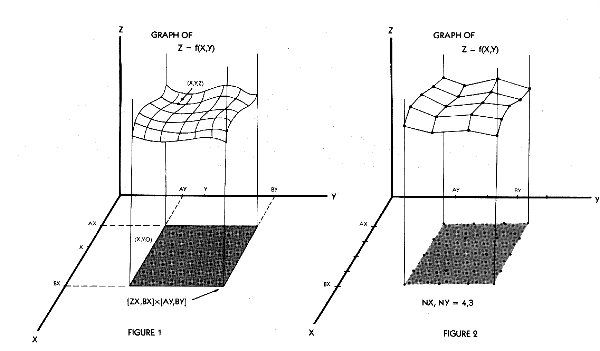FUNCTIONS TO TRY
To get you started here's a list of functions with suggested rectangular domain and eye location. When you build up the courage to try your own just be careful to avoid the "value error" trap. Be sure that the function you use is defined on the entire rectangular region. Graph 3D will accept each of the following examples. Use the menu selections [F], [I], and [E] to input the data.

1. Z=1-2*X*X
[-2,2]x[-1 ,1] eye:1 0,10,5

2. Z=X*X+2*y*y
[-1,1]x[-1,1] eye:10,14 4

3. Z=SIN(3*X)
[-1,1]x[-1,1] eye:10,15,12

4. Z=SQR(X*X+3*Y*Y)
[-3,3]x[-1 ,l] eye:1 0,2,3

5. Z=EXP(-X*X-Y*Y)
[-2,2]x[-1 ,1] eye:4,10,3

6. Z=4*X*X*X*X-4*X*X+y*y
[-1,1 ]x[-1 ,1] eye:1 0,17,4

7. Z=1/(0.5+X*X+Y*Y)-1/(0.5+X*X+(Y-2)*(y-2))
[-2,2]x[-2,4] eye:20,1 0,10

8. Z=COS(3*X*Y)
[-1 ,1 ]x[-2,2] eye:20,1 0,40

9. EXP(SIN(X*Y))
[-3,3]x[-3,3] eye:1 0,20,30

10. Z=X*X*X+2*Y*y*y
[-1,1]x[-1,1] eye:10,10,5

You'll find the 3D graphs of some of these functions illustrating the article. If you create an impressive new function, be sure to send it to Antic as an I/O Board letter.

Paul Chabot, professor of mathematics and computer science at California State University, Los Angeles, returns to BASIC here after publishing ACTION! graphics programs in the April and June, 1985 issues of Antic.